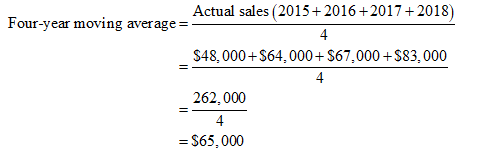# This a 4 part question that am confused about. Please show the work by hand not excel.  4-1 Sales of a particular product (in the thousands of dollars) for the years 2015 through 2018 have been \$48,000, \$64,000, \$67,000 and \$83,000 respectively. (a)What sales would you predict for 2019, using simple four-year moving avaerage? (b) What sales would you predict for 2019, using a weighted moving average with weights of 0.50 for the immediate preceding year and 0.3, 0.15, and 0.05 for the three years before that? (I am able to calculate and know the answer for (a) \$65,000 and (b) \$73,000.     What I don't understand is the following :    4-2 Using exponential smoothing with a weight of 0.6 on actual values: (a) If sales are \$45,000 and \$50,000 for 2017 and 2018, what would you forecast for 2019 ? (The first forecast is equal to the value of the preceding year.)  (b) Given this forecast and actual 2019 sales of \$53,000, what would you then forecast for 2020?   4-3 In Problem 4-1, taking actual 2015 sales of \$48,000bas the forcast for 2016, what sales would you for cast for 2017, 2018, and 2019, using exponential smoothing and a weight on actual values of (a) 0.4 and (b) 0.8 ?    Finally  4-4 In Problem 4-1, what sales would you forecast for 2019, using the simple regression (least squares) method ?

Question
72 views

This a 4 part question that am confused about. Please show the work by hand not excel.  4-1 Sales of a particular product (in the thousands of dollars) for the years 2015 through 2018 have been \$48,000, \$64,000, \$67,000 and \$83,000 respectively. (a)What sales would you predict for 2019, using simple four-year moving avaerage? (b) What sales would you predict for 2019, using a weighted moving average with weights of 0.50 for the immediate preceding year and 0.3, 0.15, and 0.05 for the three years before that? (I am able to calculate and know the answer for (a) \$65,000 and (b) \$73,000.     What I don't understand is the following :    4-2 Using exponential smoothing with a weight of 0.6 on actual values: (a) If sales are \$45,000 and \$50,000 for 2017 and 2018, what would you forecast for 2019 ? (The first forecast is equal to the value of the preceding year.)  (b) Given this forecast and actual 2019 sales of \$53,000, what would you then forecast for 2020?   4-3 In Problem 4-1, taking actual 2015 sales of \$48,000bas the forcast for 2016, what sales would you for cast for 2017, 2018, and 2019, using exponential smoothing and a weight on actual values of (a) 0.4 and (b) 0.8 ?    Finally  4-4 In Problem 4-1, what sales would you forecast for 2019, using the simple regression (least squares) method ?

check_circle

a) Determine the sales in 2019 using four-year moving average:

Note: I have answered for 4-1 only. Kindly post the other question separately.Hence, the sales in 2019 is \$65,000 according to four-year moving average.

...

### Want to see the full answer?

See Solution

#### Want to see this answer and more?

Solutions are written by subject experts who are available 24/7. Questions are typically answered within 1 hour.*

See Solution
*Response times may vary by subject and question.
Tagged in